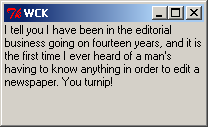back

# In Progress: Displaying Formatted Text (Writing Widgets in Python, Part 5)

June 2003 | Fredrik Lundh

The Widget Construction Kit (WCK) is a programming interface that you can use to create new widgets for Tkinter and other toolkits, in pure Python.

This is the fifth article in a series. In this article, we’ll look at how to display nicely formatted text in a WCK widget.

:::

Not much here yet!

## Formatting Text Paragraphs

```from WCK import Widget, FONT, FOREGROUND

class SimpleTextView(Widget):

ui_option_text = ""

ui_option_width = 200
ui_option_height = 100

ui_option_font = FONT

ui_option_foreground = FOREGROUND

def ui_handle_config(self):
self.font = self.ui_font(
self.ui_option_foreground, self.ui_option_font
)
return int(self.ui_option_width), int(self.ui_option_height)

def ui_handle_repair(self, draw, x0, y0, x1, y1):
space = draw.textsize(" ", self.font)
words = self.ui_option_text.split()
x = y = 0
for word in words:
# check size of this word
w, h = draw.textsize(word, self.font)
# figure out where to draw it
if x:
x += space
if x + w > x1:
# new line
x = 0
y += h
draw.text((x, y), word, self.font)
x += w```

The drawing code treats the text as a single paragraph, consisting of one long list of words. Note that the code always draws words that start at the left margin, even if they won’t fit on the line. For all other words (where x is not zero), the code checks if there’s room for a space character and the word on the current line. If not (that is, if x+w>x1 where x1 is the right margin), it moves on to the next line.

Here’s an example:

```from Tkinter import *

root = Tk()

TEXT = """
I tell you I have been in the editorial business going on fourteen
years, and it is the first time I ever heard of a man's having to know
anything in order to edit a newspaper. You turnip!
"""

text = SimpleTextView(root, text=TEXT)
text.pack(fill=BOTH, expand=1)

mainloop()```## Speeding Things Up

A little refactoring:

```from WCK import Widget, FONT, FOREGROUND

class SimpleTextView(Widget):

ui_option_text = ""

ui_option_width = 200
ui_option_height = 100

ui_option_font = FONT

ui_option_foreground = FOREGROUND

def __init__(self, master, **options):
self.width = None
self.words = None
self.ui_init(master, options)

def ui_handle_resize(self, width, height):
if width != self.width:
self.width = width
self.words = None # force reflow

def ui_handle_config(self):
self.font = self.ui_font(
self.ui_option_foreground, self.ui_option_font
)
self.words = None
return int(self.ui_option_width), int(self.ui_option_height)

def ui_handle_repair(self, draw, x0, y0, x1, y1):
if self.words is None:
# reflow the text
if not self.width:
return
self.words = []
x = y = 0
f = self.font
space = draw.textsize(" ", f)
for word in self.ui_option_text.split():
w, h = draw.textsize(word, f)
if x:
x += space
if x + w > self.width:
x = 0
y += h
self.words.append(((x, y), word, f))
x += w
# render
text = draw.text
for args in self.words:
text(*args)```

## Displaying Scrolling Pagesrendered by a django application. hosted by webfaction.